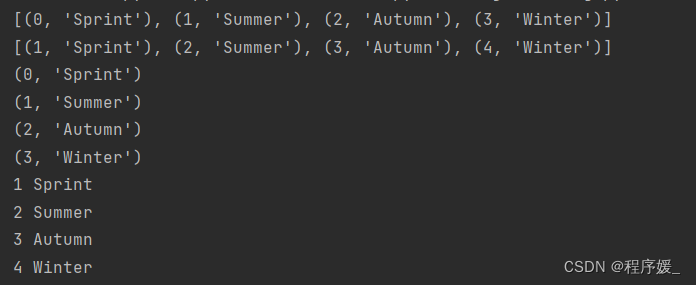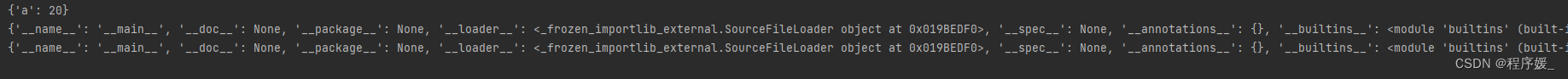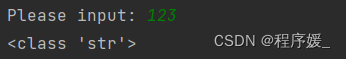## 【Python】内置函数

article2023/9/27 10:44:11/

### 文章目录

• 反射相关【4】
• 基础数据类型相关【38】
• 和数字相关（14）
• 数据类型 <4>
• ==bool([x])==
• ==int((x, base=10)==
• ==float([x])==
• ==complex([real[, imag]])==
• 进制转换 <3>
• ==bin(x)==
• ==oct(x)==
• ==hex(x)==
• 数学运算（7）
• ==abs(x)==
• ==divmod(a, b)==
• ==round(x [, n] )==
• ==pow(x, y[, z])==
• ==sum(iterable[, start])==
• ==min( x, y, z, .... )==
• ==max( x, y, z, .... )==
• 和数据结构相关（24）
• 序列 <13>
• 列表和元组 2
• ==list(tup)==
• ==tuple(iterable)==
• 相关内置函数 2
• ==reversed(list)==
• ==slice(start, stop[, step])==
• 字符串 9
• ==str()==
• ==format()==
• ==bytes== 【已废弃】
• ==bytearry==
• ==memoryview(obj)==
• ==ord\(c\)==
• ==chr(i)==
• ==ascii\(c\)==
• ==repr(object)==
• 数据集合 <3>
• 字典 1
• ==dict()==
• 集合 2
• ==set([iterable])==
• ==frozenset([iterable])==
• 相关内置函数 <8>
• ==len(s)==
• ==sorted(iterable, key=None, reverse=False)==
• ==enumerate(sequence, [start=0])==
• ==all(iterable)==
• ==any(iterable)==
• ==zip([iterable, ...])==
• ==filter(function, iterable)==
• ==map(function, iterable, ...)==
• 作用域相关【2】
• ==locals()==
• ==globals()==
• 面向对象相关【9】
• 迭代器/生成器相关【3】
• ==range(start, stop[, step])==
• ==next(iterable[, default])==
• ==iter(object[, sentinel])==
• 其他【12】
• 字符串类型代码的执行（3）
• ==eval(expression[, globals[, locals]])==
• ==exec(expression)==
• ==compile(source, filename, mode[, flags[, dont_inherit]])==
• 输入输出（2）
• ==input([prompt])==
• ==print(*objects, sep=' ', end='\n', file=sys.stdout, flush=False)==
• 内存相关（2）
• ==hash(object)==
• ==id([object])==
• 文件操作相关（1）
• ==open("filePah", mode="", encoding="utf-8")==
• 模块相关（1）
• ==\__import__(moduleName)==
• 帮助（1）
• ==help([object])==
• 调用相关（1）
• ==callable(object)==
• 查看内置属性（1）
• ==dir([object])==

# 基础数据类型相关【38】

## 和数字相关（14）

### 数据类型 <4>

#### bool([x])

``````print(bool())  # False
print(bool(0)) # False
print(bool(1)) # True
``````

#### int((x, base=10)

• x – 字符串或数字。
• base – 进制数，默认十进制。
``````print(int())           # 0 不传入参数，得到结果0
print(int(3.6))        # 3
print(int('12', 16))   # 18 如果是带参数base的话，12要以字符串的形式进行输入，12为16进制
print(int('0xa', 16))  # 10
``````

#### float([x])

``````print(float(1))         # 1.0
print(float(-1.5))      # -1.5
print(float('123.35'))  # 123.35
``````

#### complex([real[, imag]])

``````print(complex(1))           # (1+0j)
print(complex(1, 2))        # (1+2j)
print(complex("1+2j"))      # (1+2j) 注意：这个地方在"+"号两边不能有空格,否则会报错
``````

### 进制转换 <3>

#### bin(x)

``````print(bin(5))   # 0b101
print(0b101)    # 5
``````

#### oct(x)

``````print(oct(9))   # 0o11
print(0o11)     # 9
``````

#### hex(x)

``````print(hex(10))  # 0xa	0-f
print(0xa)      # 10
``````

### 数学运算（7）

#### abs(x)

``````print(abs(-5.5))    # 绝对值 5.5
``````

#### divmod(a, b)

``````print(divmod(10, 3))    # (3, 1) 除法 （商，余数）
``````

#### round(x [, n] )

``````print(round(9.12))          # 9
print(round(-100.965, 2))   # -100.97
``````

#### pow(x, y[, z])

``````print(pow(2, 3))    # 8
print(pow(2, 3, 5))    # 3
print(2**3)         # 8

# 以下是 math 模块 pow() 方法的语法:
import math
print(math.pow(2,3))    # 8.0
``````

#### sum(iterable[, start])

• iterable – 可迭代对象，如：列表、元组、集合。
• start – 指定相加的参数，如果没有设置这个值，默认为0。
``````print(sum([0, 1, 2]))       # 3
print(sum([0, 1, 2], 5))    # 8  列表计算总和后再加5
``````

#### min( x, y, z, … )

``````print(min(1, 0, 2))     # 0
print(min([1, 0, 2]))   # 0
print(min('012'))       # 0
``````

#### max( x, y, z, … )

``````print(max(1, 0, 2))     # 2
print(max([1, 0, 2]))   # 2
print(max('012'))       # 2
``````

## 和数据结构相关（24）

### 序列 <13>

#### 列表和元组 2

##### list(tup)

``````print(list((1, 'a', 'b')))      # [1, 'a', 'b']
``````
##### tuple(iterable)

``````print(tuple([1, 'a', 'b']))     # ( 1, 'a', 'b')
print(tuple('abc'))             # ('a', 'b', 'c')
``````

#### 相关内置函数 2

##### reversed(list)

``````lst = [123, 'b', 'a']
rlst = reversed(lst)
print(list(rlst))		# ['a', 'b', 123]
``````
##### slice(start, stop[, step])

``````s = '今天天气不好'
s1 = '明天天气很差'
ss = slice(1, 6, 2)
print(s[ss])               # 天气好
print(s1[ss])              # 天气差
``````

#### 字符串 9

##### format()
``````# 字符串
print(format('test', '<20'))    # 左对齐 test
print(format('test', '>20'))    # 右对齐                 test
print(format('test', '^20'))    # 居中         test

# 数值
print(format(3, 'b'))   # 二进制 11
print(format(97, 'c'))   # 转换成unicode字符 a
print(format(11, 'd'))   # 十进制 11
print(format(11, 'o'))   # 八进制 13
print(format(11, 'x'))   # 十六进制(小写字母） b
print(format(11, 'X'))   # 十六进制（大写字母） B
print(format(48, '08d'))    # 00000048
print(format(11, 'n'))   # 和d一样 11
print(format(11))        # 和d一样 11

# 浮点数
print(format(123456789, 'e'))   # 科学计数法，默认保留6位小数 1.234568e+08
print(format(123456789, '0.2e'))   # 科学计数法，保留2位小数（小写） 1.23e+08
print(format(123456789, '0.2E'))   # 科学计数法，保留2位小数（大写） 1.23E+08
print(format(1.23456789, 'f'))   # 小数点计数法，保留6位小数 1.234568
print(format(1.23456789, '0.2f'))   # 小数点计数法，保留2位小数 1.23
print(format(1.23456789, '0.10f'))   # 小数点计数法，保留10位小数 1.2345678900
``````
``````print("{} {}".format("hello", "python"))     # hello python 不设置指定位置，按默认顺序
print("{1} {0}".format("python", "hello"))     # hello python 设置指定位置
# 通过字典设置参数
dict = {"name": "百度", "url": "www.baidu.com"}
print("网站名：{name}, 地址：{url}".format(**dict))    # 网站名：百度, 地址：www.baidu.com
# 通过列表索引设置参数
lst = ['百度', 'www.baidu.com']
print("网站名：{0}, 地址：{0}".format(lst))  # 网站名：百度, 地址：www.baidu.com       0是必须的！！
``````
##### bytearry

``````print(bytearray([1,2,3]))   # bytearray(b'\x01\x02\x03')
``````
##### memoryview(obj)

``````mv = memoryview(bytearray('abcd', 'utf-8'))
print(mv)
print(mv[0:2].tobytes())    # b'ab'
``````
##### ord(c)

``````print(ord('中'))     # 20013
print(ord('a'))      # 97
``````
##### chr(i)

``````print(chr(20013))     # 中 十进制
print(chr(0x61))      # a 十六进制

for i in range(32, 126):     # 第 33～126 号（共94个）是字符，其中第 48～57 号为 0～9 十个阿拉伯数字，65～90 号为 26 个大写英文字母，97～122 号为 26 个小写英文字母，其余为一些标点符号、运算符号等。
print(chr(i), end=' ')
``````
##### ascii(c)
``````print(ascii('中'))		#'\u4e2d'
``````
##### repr(object)

``````print(str("你好，我叫\t'Jessica'"))        # 你好，我叫'Jessica'
print(repr("你好，我叫\t'Jessica'"))       # "你好，我叫'Jessica'"
``````

### 数据集合 <3>

#### 字典 1

##### dict()

``````d1 = dict()
print(d1)       # 空字典 {}

d2 = dict(a=1, b=2, c=3)
print(d2)       # 传入关键字 {'a': 1, 'b': 2, 'c': 3}

d3 = dict([('a', 1), ('b', 2), ('c', 3)])
print(d3)       # 可迭代对象方式来构造字典 {'a': 1, 'b': 2, 'c': 3}

d4 = dict(zip(['a', 'b', 'c'], [1, 2, 3]))
print(d4)       # 映射函数方式来构造字典 {'a': 1, 'b': 2, 'c': 3}
``````

#### 集合 2

• list vs tuple
list:：有序，可重复，可变序列
tuple：有序，可重复，不可变序列（只读）
• set vs frozenset
set：无序，不可重复，可变序列
frozenset：无序，不可重复，不可变序列（只读）
##### set([iterable])

``````x = set('hello')
y = set('python')
print(x, y)         # {'e', 'o', 'h', 'l'} {'t', 'y', 'p', 'o', 'h', 'n'}   重复的被删除
print(x & y)        # {'o', 'h'} 交集
print(x | y)        # {'y', 'h', 'e', 'p', 'l', 'n', 't', 'o'} 并集
print(x - y)        # {'l', 'e'} 差集
``````
##### frozenset([iterable])

``````s = frozenset(range(3))
print(s)        # frozenset({0, 1, 2})
s2 = frozenset([1, 1, 2, 3])
print(s2)       # frozenset({1, 2, 3})
``````

### 相关内置函数 <8>

#### len(s)

``````print(len('hello'))  # 5 字符串长度
print(len([1, 2, 3, 4, 5]))  # 5 列表元素个数
``````

#### sorted(iterable, key=None, reverse=False)

sort 与 sorted 区别：
sort 是应用在 list 上的方法，sorted 可以对所有可迭代的对象进行排序操作。
list 的 sort 方法返回的是对已经存在的列表进行操作，无返回值，而内建函数 sorted 方法返回的是一个新的 list，而不是在原来的基础上进行的操作。

``````lst = ['john', 'ken', 'jessica']
# 按名字长度排序
new_lst = sorted(lst, key=lambda item: len(item))
print(new_lst)      # ['ken', 'john', 'jessica']

lst = [('john', 'm', 15), ('ken', 'm', 20), ('jessica', 'f', 18)]
# 按年龄降序排序
new_lst = sorted(lst, key=lambda l:l, reverse=True)
print(new_lst)      # [('ken', 'm', 20), ('jessica', 'f', 18), ('john', 'm', 15)]

lst = [{'name': 'john', 'age': 15}, {'name': 'ken', 'age': 20}, {'name': 'jessica', 'age': 18}]
# 按年龄排序
new_lst = sorted(dict, key=lambda dict: dict['age'])
print(new_lst)  # [{'name': 'john', 'age': 15}, {'name': 'jessica', 'age': 18}, {'name': 'ken', 'age': 20}]
``````

#### enumerate(sequence, [start=0])

• sequence – 一个序列、迭代器或其他支持迭代对象。
• start – 下标起始位置的值。
``````seasons = ['Sprint', 'Summer', 'Autumn', 'Winter']
print(list(enumerate(seasons)))             # [(0, 'Sprint'), (1, 'Summer'), (2, 'Autumn'), (3, 'Winter')]
print(list(enumerate(seasons, start=1)))    # [(1, 'Sprint'), (2, 'Summer'), (3, 'Autumn'), (4, 'Winter')]  下标从1开始

for item in enumerate(seasons):
print(item)

for i, item in enumerate(seasons, start=1):
print(i, item)
``````#### all(iterable)

``````print(all(['a', 'b', 'c']))     # True
print(all(['a', '', 'c']))      # False 存在一个为空的元素
print(all([0, 1, 2, 3]))        # False 存在一个未0的元素
``````

#### any(iterable)

``````print(any(['a', 'b', 'c', '']))             # True
print(any(['', 0, False, [], (), {}]))      # False 存在一个为空的元素
``````

#### zip([iterable, …])

``````lst1 = [1, 2, 3]
lst2 = ['a', 'b', 'c', 'd']
z = zip(lst1, lst2)
print(list(z))      # [(1, 'a'), (2, 'b'), (3, 'c')] 元素个数与最短的列表保持一致

l1, l2 = zip(*zip(lst1, lst2))      # 与zip相反，zip(*)可理解为解压，返回二维矩阵式
print(list(l1))     # [1, 2, 3]
print(list(l2))     # ['a', 'b', 'c']
``````

#### filter(function, iterable)

``````# 过滤列表中的奇数
odd_filter = filter(lambda x: x % 2 == 1, range(1, 10))
print(list(odd_filter))  # [1, 3, 5, 7, 9]

# 筛选年龄大于18的数据
lst = [{'name': 'june', 'age': 27},
{'name': 'ken', 'age': 39},
{'name': 'bob', 'age': 10}]

age_filter = filter(lambda dict: dict['age'] > 18, lst)
print(list(age_filter))     # [{'name': 'june', 'age': 27}, {'name': 'ken', 'age': 39}]
``````

#### map(function, iterable, …)

``````# 计算列表中每个元素的平方
mp = map(lambda x: x ** 2, range(1, 10, 2))
print(list(mp))  # [1, 9, 25, 49, 81]
# 等价于：
print([i**2 for i in range(1, 10, 2)])      # [1, 9, 25, 49, 81]

# 计算2个列表中相同位置的数据的和
lst1 = [1, 2, 3, 4, 5]
lst2 = [6, 7, 8, 9, 10]
mp = map(lambda x, y: x + y, lst1, lst2)
print(list(mp))     # [7, 9, 11, 13, 15]
``````

# 作用域相关【2】

## globals()

``````a = 10
def func():
a = 20
print(locals())

func()
print(globals())	# 查看全局作用域所有内容
print(locals())		# 查看当前作用域中的内容
``````# 迭代器/生成器相关【3】

## range(start, stop[, step])

``````for i in range(10):
print(i)
``````

## next(iterable[, default])

• iterable – 可迭代对象
• default – 可选，用于设置在没有下一个元素时返回该默认值，如果不设置，又没有下一个元素则会触发 StopIteration 异常。

## iter(object[, sentinel])

• object – 支持迭代的集合对象。
• sentinel – 如果传递了第二个参数，则参数 object 必须是一个可调用的对象（如，函数），此时，iter 创建了一个迭代器对象，每次调用这个迭代器对象的__next__()方法时，都会调用 object。
``````lst = [1, 2, 3]
it = lst.__iter__()
print(it.__next__())     # 1
it2 = iter(lst)          # 等价于：lst.__iter__()
print(next(it2))         # 1 等价于：lst__next__()

# def iter(obj):
#     return obj.__iter__()
#
# def next(obj):
#     return obj.__next__()
``````

# 其他【12】

## 字符串类型代码的执行（3）

### eval(expression[, globals[, locals]])

• expression – 表达式。
• globals – 变量作用域，全局命名空间，如果被提供，则必须是一个字典对象。
• locals – 变量作用域，局部命名空间，如果被提供，可以是任何映射对象。
``````r = eval('1+1')     # 可以把字符串当成代码去执行，有返回值
print(r)    # 2
``````

### exec(expression)

``````# 单行语句字符串
exec('print("Hello Python")')	# Hello Python

# 多行语句字符串
exec("""
for i in range(5):
print(i)
""")

'''
# 结果如下：
0
1
2
3
4
'''
``````

### compile(source, filename, mode[, flags[, dont_inherit]])

• source – 字符串或者AST（Abstract Syntax Trees）对象。。
• filename – 代码文件名称，如果不是从文件读取代码则传递一些可辨认的值。
• mode – 指定编译代码的种类。可以指定为 exec, eval, single。
• flags – 变量作用域，局部命名空间，如果被提供，可以是任何映射对象。
• flags和dont_inherit是用来控制编译源码时的标志。
``````str = "for i in range(1, 3): print(i)"
c = compile(str, '', 'exec')    # 编译成字节代码对象
exec(c)	# 1 2

str = "3 * 4 + 5"
r = compile(str, '', 'eval')
print(eval(r))      # 17
``````

## 输入输出（2）

### input([prompt])

``````a = input('Please input: ')
print(type(a))
``````### print(*objects, sep=’ ‘, end=’\n’, file=sys.stdout, flush=False)

• objects – 复数，表示可以一次输出多个对象。输出多个对象时，需要用 , 分隔。
• sep – 用来间隔多个对象，默认值是一个空格。
• end – 用来设定以什么结尾。默认值是换行符 \n，我们可以换成其他字符串。
• file – 要写入的文件对象。
• flush – 输出是否被缓存通常决定于 file，但如果 flush 关键字参数为 True，流会被强制刷新。
``````print("www","baidu","com",sep=".")  # 设置间隔符 www.baidu.com
``````

## 内存相关（2）

### hash(object)

** 定义：** 返回对象的哈希值。

``````print(hash('呵呵'), hash('哈哈'))	# -855838824 538020339 （每次运行值都不同）
``````

### id([object])

``````lst1 = [1, 2, 3]
lst2 = [1, 2, 3]
print(id(lst1), id(lst2))   # 20858360 20859520 （每次运行值都不同）
``````

## 文件操作相关（1）

### open(“filePah”, mode=“”, encoding=“utf-8”)

``````f = open("xxx", mode="", encoding="utf-8")
f.rea()
for line in f:
pass

f.write()

with open() as f:
pass
``````

## 模块相关（1）

### _import_(moduleName)

``````# 已知导入模块：
import os
# 未知，输入导入模块：
mo = input('>>>')   # "os"
__import__(mo)      # 动态加载一个模块
``````

## 帮助（1）

### help([object])

``````print(help(str))	# 查看 str 数据类型的帮助
``````

## 调用相关（1）

### callable(object)

``````def func():
pass

print(callable(func))   # True
print(callable(123))    # False
``````
``````def run():
print('I can run.')

def func(fn):
if callable(fn):    # 判断该内容是否可以被调用
fn()
else:
print('The input func can not callable.')

func(run)	# I can run.
func(123)	# The input func can not callable.
``````

## 查看内置属性（1）

### dir([object])

``````print(dir())
# ['__builtins__', '__doc__', '__file__', '__loader__', '__name__', '__package__', '__spec__']
print(dir(str))
# ['__add__', '__class__', '__contains__', '__delattr__', '__dir__', '__doc__', '__eq__', '__format__', '__ge__', '__getattribute__', '__getitem__', '__getnewargs__', '__gt__', '__hash__', '__init__', '__init_subclass__', '__iter__', '__le__', '__len__', '__lt__', '__mod__', '__mul__', '__ne__', '__new__', '__reduce__', '__reduce_ex__', '__repr__', '__rmod__', '__rmul__', '__setattr__', '__sizeof__', '__str__', '__subclasshook__', 'capitalize', 'casefold', 'center', 'count', 'encode', 'endswith', 'expandtabs', 'find', 'format', 'format_map', 'index', 'isalnum', 'isalpha', 'isascii', 'isdecimal', 'isdigit', 'isidentifier', 'islower', 'isnumeric', 'isprintable', 'isspace', 'istitle', 'isupper', 'join', 'ljust', 'lower', 'lstrip', 'maketrans', 'partition', 'replace', 'rfind', 'rindex', 'rjust', 'rpartition', 'rsplit', 'rstrip', 'split', 'splitlines', 'startswith', 'strip', 'swapcase', 'title', 'translate', 'upper', 'zfill']
``````

### 报表控件FastReport使用指南——使用NuGet包创建PDF文档

FastReport 是功能齐全的报表控件&#xff0c;可以帮助开发者可以快速并高效地为.NET&#xff0c;VCL&#xff0c;COM&#xff0c;ActiveX应用程序添加报表支持&#xff0c;由于其独特的编程原则&#xff0c;现在已经成为了Delphi平台最优秀的报表控件&#xff0c;支持将编程开…

### Python3中goto的用法

Python3代码指定跳转可以使用goto这个库&#xff1a; 安装&#xff1a; pip install goto-statement 一般安装的版本是1.2 需要做以下修改才能正常使用&#xff1a; python 使用goto&#xff0c;遇到的问题解决_奶嘴偷走初吻的博客-CSDN博客python goto 出现报错:Attribut…

### 类的成员之：构造器(构造方法)

1.构造器的特征&#xff1a; 它具有与类相同的名称它不声明返回值类型。&#xff08;与声明为void不同&#xff09;不能被static、final、synchronized、abstract、native修饰&#xff0c;不能有return语句返回值 2.构造器的作用&#xff1a; 1.创建对象2.初始化对象的…

### linux环境搭建

&#x1f388;个人主页:&#x1f388; :✨✨✨初阶牛✨✨✨ &#x1f43b;推荐专栏: &#x1f354;&#x1f35f;&#x1f32f;C语言进阶 &#x1f511;个人信条: &#x1f335;知行合一 &#x1f349;本篇简介:>:介绍学习如何使用云服务器搭建Linux的环境. 前言 linux介绍…

### Linux系统之编译安装python3

Linux系统之编译安装python3 一、python3介绍1. python3简介2. python3特点 二、检查本地环境1. 检查本地操作系统版本2. 检查内核版本3. 检查当前python版本 三、安装前准备工作四、下载python最新版本源码包1. 访问python官网2. 创建下载目录3. 下载python源码包4. 解压pytho…

### Redis 常见面试题

1. 认识Redis Redis是一个开源的内存数据结构存储&#xff0c;Redis是一个基于内存的数据库&#xff0c;对数据的读写都在内存中完成&#xff0c;因此数据读写速度非常快&#xff0c;常用于缓存&#xff0c;分布式锁等&#xff0c;MySQL的表数据都存储在 t_order.ibd&#xff…

### Java之旅（三）

Java 输出&#xff1a;println()、print() 使用 println() 方法在 Java 中输出值或打印文本。 System.out.println("Hello World!"); println() 可以根据需要添加任意数量的方法。请注意&#xff0c;它将为每个方法添加一个新行&#xff1a; System.out.println(&…

### Unity2D骨骼动画制作之单张图片编辑

1、打开骨骼制作面板 在Sprite Editor左侧选项&#xff0c;选择Skinning Editor 2、 &#xff08;1&#xff09;骨骼制作 Preview Pose 预览模式&#xff0c;可以预览动作并不会真正的改变设置 Reset Pose 将角色骨骼和关节恢复到原始位置 Edit Bone 编辑骨骼&#xff0c;…